# Induced map on spectra by a ring homomorphism

Suppose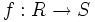$f:R \to S$ is a homomorphism of commutative unital rings. Then, if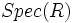$Spec(R)$ and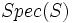$Spec(S)$ denote the spectra of the rings$R$ and$S$ respectively, we have a functorially induced mapping: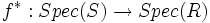$f^*: Spec(S) \to Spec(R)$

sending a prime ideal of$S$ to its contraction in$R$. The well-definedness of this map rests on the fact that the contraction of a prime ideal is prime.

Various ring-theoretic assumptions on the nature of$f$ give set-theoretic or topological information about the map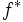$f^*$.

## Surjective homomorphism yields injective map

If$f:R \to S$ is surjective, the map$f^*: Spec(S) \to Spec(R)$ is injective. In fact,$Spec(S)$ is identified with those elements of$Spec(R)$ that contain the kernel of$f$.

Thus, the map is injective and its image is a closed subset. In fact, the map is a closed map with respect to the topologies on both sides.

Intuitively, what is happening is that when we are quotienting out by an ideal, we are retaining only those prime ideals which contain that ideal.

This can be better understood in relation with the Galois correspondence between a ring and its spectrum.

## Localization yields injective map

Further information: map to localization is injective on spectra If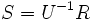$S = U^{-1}R$ for some multiplicative subset$U$ of$R$, and$f$ is the natural map from$R$ to$S$, then$f^*$ is again an injective map, but this time the image is not necessarily a closed set.$f^*$ identifies$Spec(S)$ with those prime ideals of$R$ that do not intersect$U$.

Intuitively, what is happening is that when we are localizing at a multiplicative subset, we are retaining only those prime ideals that are disjoint from that multiplicative subset.

## Finite morphism yields map with finite fibers

Further information: finite morphism implies finite on spectra

If$f$ is a finite morphism, in the sense that$S$ is a finitely generated module over$R$, then the map$f$ has finite fibers. In fact, the sizes of the fibers are bounded from above by the rank of$S$ as a$R$-module. This somehow indicates that the size of the fibers measures the module-theoretic dimension of$S$ over$R$.

## Integral extension yields surjective map

Further information: integral extension implies surjective on spectra

If$f$ is injective and an integral morphism i.e. if$S$ is an integral extension of$R$, the induced map$f^*$ is surjective. In special situations like a ring of integers, there is a more concrete interpretation of this: any prime ideal in the smaller ring, splits as a product of prime ideals in the bigger ring, and these factors are the fibers of the prime ideal we started with. In general, the picture is more complicated.